•5星
2.28MB qq_40957277 2021-06-22 18:53:14
• Python聚类分析 Python3星
64KB u010154424 2016-08-13 09:14:53
• 266KB weixin_38538021 2020-09-16 18:52:20
• 5KB qq_42617330 2018-07-23 21:45:15
• 59KB weixin_38582506 2020-09-21 19:13:18
• 1.31MB weixin_38617602 2021-01-20 02:57:30
• 本文是萝卜的Python数据挖掘实战的第7篇1 - 基于不平衡数据的反欺诈模型实战2 - Apriori算法实现智能推荐3- 随机森林预测宽带客户离网4 - 多元线性回归模型实战5 -...本文是萝卜的Python数据挖掘实战的第7篇

01

前言

聚类分析是研究分类问题的分析方法，是洞察用户偏好和做用户画像的利器之一，也可作为其他数据分析任务的前置探索(如EDA)。上文的层次聚类算法在数据挖掘中其实并不常用，因为只是适用于小数据。所以我们引出了 K-Means 聚类法，这种方法计算量比较小。能够理解 K-Means 的基本原理并将代码用于实际业务案例是本文的目标。下文将详细介绍如何利用 Python 实现基于 K-Means 聚类的客户分群，主要分为两个部分：

• 详细原理介绍

• Python代码实战

02

原理介绍

上一篇层次聚类的推文中提到「既然它们能被看成是一类的，所以要么它们距离近，要么它们或多或少有共同的特征」。为了能够更好地深入浅出，我们像上次那样调整一下学习顺序，将数学公式往后放，先从聚类过程与结果入手。注意，本文先以样本之间的距离为聚类指标。

K-Means 聚类的目标就一句话「将 n 个观测数据点按照一定标准划分到 k 个聚类中」。至于这个标准怎么定夺以及如何判断聚类结果好坏等问题，文章后半段会提及。

K-Means 聚类的步骤用这一张图就可以表达出来。（这里的 k 为 2，即分成两类）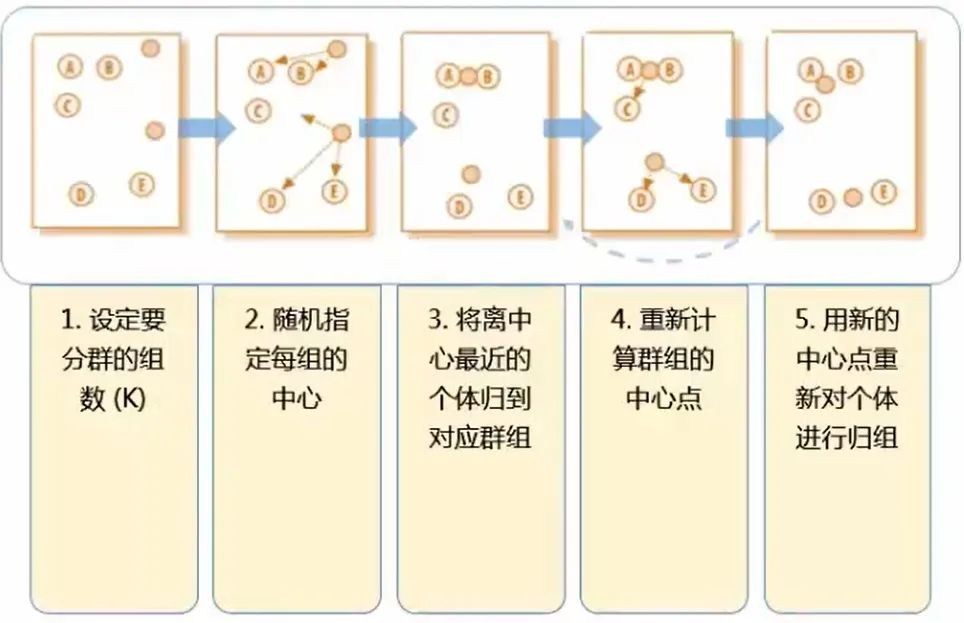2.1 关于kmeans的一些问题

问：在第二步的随机指定每组的中心 这个步骤中，明摆着 ABC 为一类，DE 为一类 才是最正确的分类方式，毕竟肉眼就可以判断距离了，为什么指定每组的中心后反倒分类错误了呢？（第二步是将 AB 一类，CDE 一类）？

答：别着急，K-Means 算法并不求一步就完全分类正确。第二步到第三步的过程被称为“中心迭代“。一开始是随机的指定每组的中心，这个中心可能是有偏颇的，所以第三步是用每个类的中心来代替第二步中随即指定的中心。接下来再计算每个点到中心的距离，就会发现 C 这个点其实是离上面的中心更近（AB 一类，DE 一类本来就分类正确了，只是 C 出现了分类失误）

问：图中经过第四步后其实就已经划分出了正确的分类，第五步还有什么用呢？

答：第四步到第五步这个过程跟第二到第三步一样，也叫 “ 中心迭代 ”，即将新分好的正确的类的中心作为群组的中心点。这样才能为下一步的分类做准备，毕竟数据量并不只是图中的五个点，这一套(五步)流程也不是只运行一次就能完成分类，需要不断重复，最终的结果便是不会再有点像 C 那样更换分类的情况。

问：第一步要计算几次距离？（k=2）答：需要计算 10 次距离，随即指定的两个中心点到每一个已知点的距离：中心1，2 分别到点 ABCDE 的距离。其实也就是 n 个点，分成 k 类时，需要计算 n×k 次。

问：第一步用层次聚类法也是 10 次，为什么还说 K-Means 是一个计算量较小的方法呢？

答：对第一步使用层次聚类法的计算次数为：Cn2（（C52 = (54)/2））即计算两两点之间的距离，然后再比较筛选，即：n(n-1) / 2 = 54 / 2 = 10 次，但这只是小的数据样本，如果样本量巨大呢（同样还是 k 为 2 时）？那么 K-Means 的 n*k 与层次聚类法的 Cn2 的复杂度图比较便是：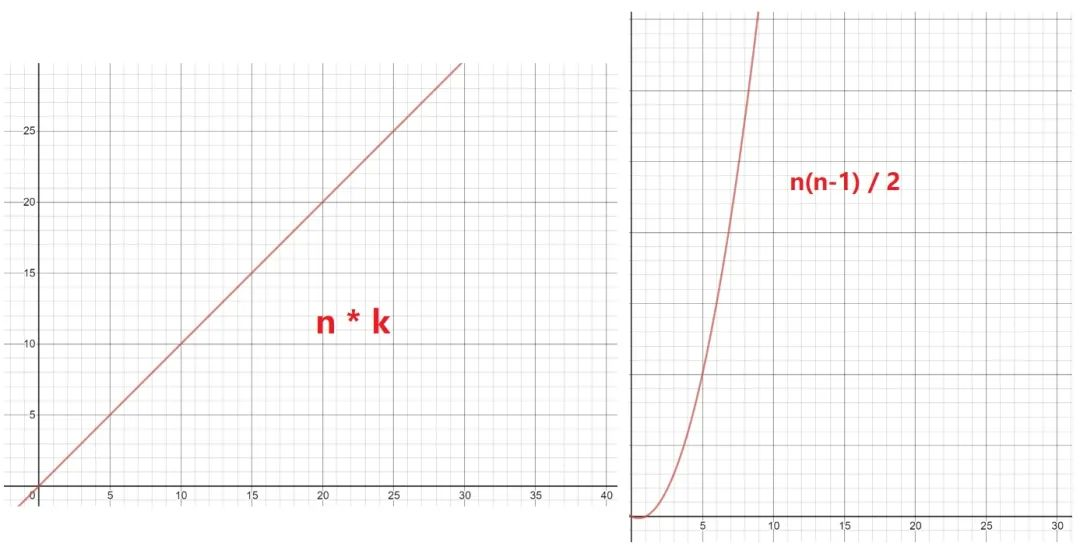数据量一旦开始增加，层级聚类法的计算复杂程度便呈指数级增长。

问：K-Means 的不足？

答：K-Means 最显著的缺点便是 k 的个数不好确定，但在商业数据挖掘上，这个缺点其实不一定难避免。因为商业数据挖掘的 k-means 聚类方法中，k 大部分都在 2 ~ 12 这个范围，所以只需要做 9 次，然后看哪种效果最好即可。

2.2 K-Means 的要点

要点1：预先处理变量的缺失值、异常值 要点2：变量标准化 要点3：不同维度的变量，相关性尽量低 要点4：如何决定合适的分群个数？·

主要推荐轮廓系数（Silhouette Coeficient），并结合以下注意事项：

• 分群结果的稳定性

• 重复多次分群，看结果是否稳定

• 分群结果是否有好解释的商业意义

也有一种相对没那么严谨的分类方法，这种方法通常会分 5~8 类，这样既能反映工作量（给领导看），又不至于太累（分出 12 类，还要对每一类的特征进行探索，描述性统计分析等）

2.3 轮廓系数最好的分类结果：不同组之间的差距越大越好，同组内的样本差距越小越好。这样才能更好的体现物以类聚的思想（e.g: 同一类人的三观非常一致，不同类的人之间三观相差甚远）。因为组内差异为零的话，a(i) 便无限接近于0，公式分子便为 b(i)，分母 max{0, b(i)} = b(i)，所以结果为 b(i) / b(i) = 1，即越趋近于 1 代表组内聚类性和组间的分离度越好。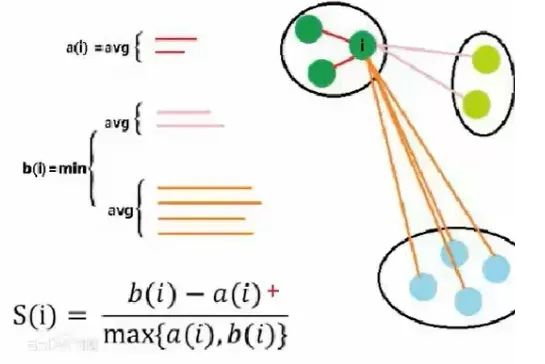轮廓系数其实非常难求，组内的(a(i))好算，b(i) 非常难计算，而且还要每个点都要同不同组里面的所有点进行计算，所以轮廓系数在实操的时候样本量的大小需要控制，一般几千就行了，几万的话就太难计算了；换言之，轮廓系数一般也是在探索的时候用，比如分层抽样后对 k 的取值进行探索。这也再次呼应了前两段提到的 K-Means 方法的 k 难以直接通过数学公式求得的的这一特点。

2.4 K-Means 聚类的两种用法

1、发现异常情况：如果不对数据进行任何形式的转换，只是经过中心标准化或级差标准化就进行快速聚类，会根据数据分布特征得到聚类结果。这种聚类会将极端数据聚为几类。这种方法适用于统计分析之前的异常值剔除，对异常行为的挖掘，比如：监控银行账户是否有洗钱行为、监控POS机是有从事套现、监控某个终端是否是电话卡养卡客户等等。

2、将个案数据做划分：出于客户细分目的的聚类分析一般希望聚类结果为大致平均的几大类，因此需要将数据进行转换比如使用原始变量的百分位秩、Turkey正态评分、对数转换等等。在这类分析中数据的具体数值并没有太多的意义，重要的是相对位置。这种方法适用场景包括客户消费行为聚类、客户积分使用行为聚类等等。

如果变量比较多比如 10 个左右，变量间的相关性又比较高，就应该做个因子分析或者稀疏主成分分析，因为 K-Means 要求不同维度的变量相关性尽量低。（本系列的推文：原理+代码｜Python基于主成分分析的客户信贷评级实战）

那如果数据右偏严重，K-Means 聚类会出现什么情况？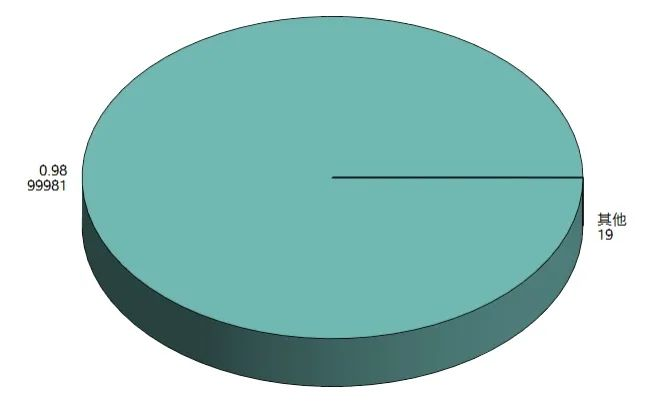如果不经过任何处理，则聚类出来的结果便是如上图那样，出现 ”绝大部分客户属于一类，很少量客户属于另外一类“ 的情况，这就失去了客户细分的意义（除非你是为了检测异常值），因为有时候我们希望客户能够被均匀的分成几类（许多领导和甲方的需求为均匀的聚类，这是出于管理的需求）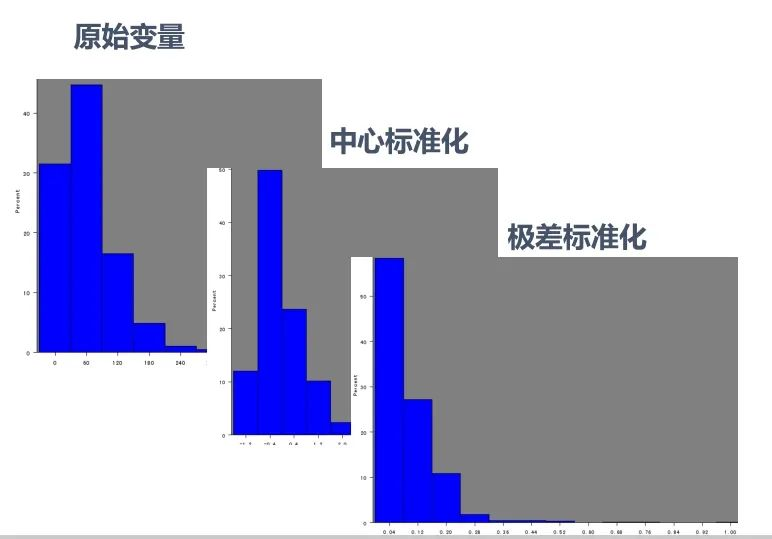原始数据本来就是右偏的，几种标准化方式之后其实也还是右偏的...，我们在学校学习统计学或聚类方法的时候，所用数据大多是来自自然科学的，所以分布情况都比较“完美”，很少出现较强的偏态分布。而现实生活与工作中的数据，如金融企业等，拿到的数据大多右偏严重。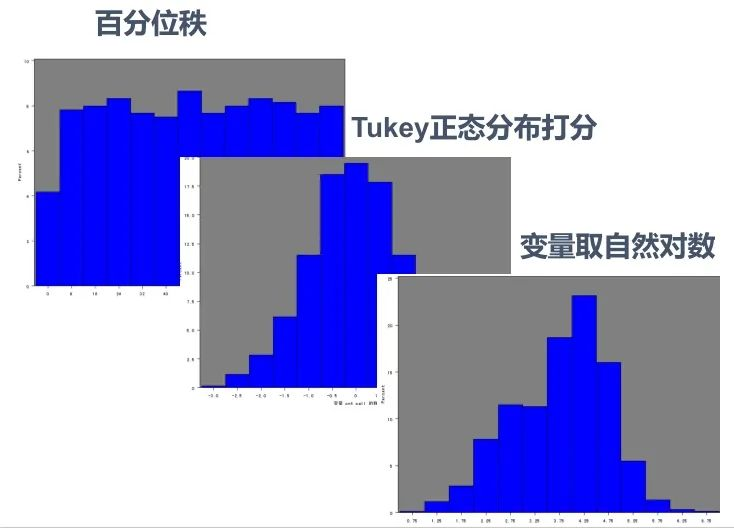上图是能够强迫将右偏数据转换成均匀分布的几种方法。但通常回归算法时的右偏处理才会使用变量取自然对数的方法，聚类算法常用 Tukey 正态分布打分的方式来处理右偏数据。

2.5 变量转换小结

非对称变量在聚类分析中选用百分位秩和Tukey正态分布打分比较多；在回归分析中取对数比较多。因为商业上的聚类模型关心的客户的排序情况，回归模型关心的是其具有经济学意义，对数表达的是百分比的变化。

2.6 使用决策树做聚类后的客户分析

聚类算法还能与决策树算法一起用（期待脸(☆▽☆)）？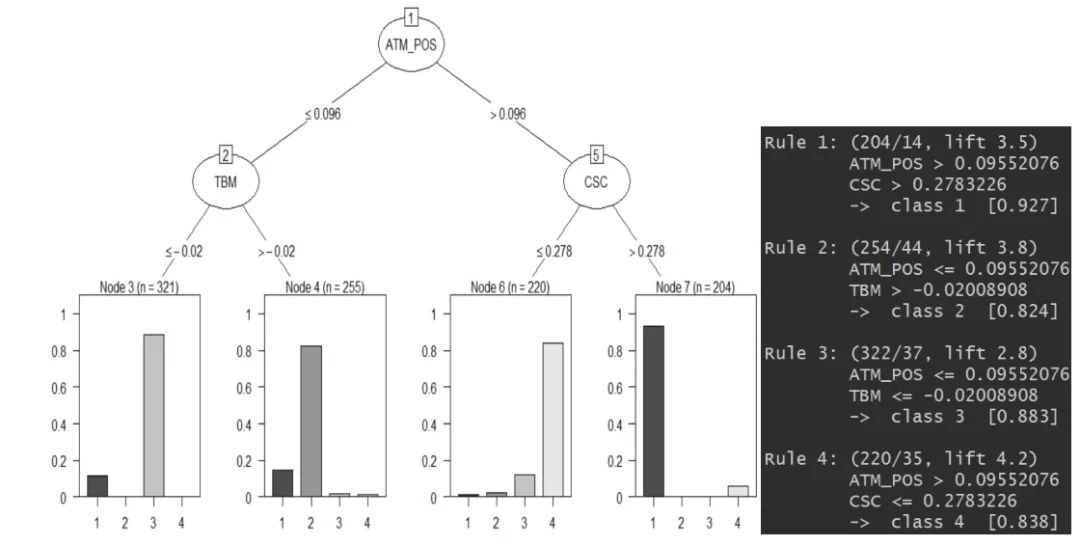轮廓系数可以为我们做 K-Means 聚类的时候提供一个 k 的参考值，而初步聚类后，我们便有了 Y，即每个数据样本所对应的类别。这时候我们画棵决策树（可以结合使用高端一些的决策树可视化方式），如果底端的叶子所呈现出的数据分类是某一类较多，其余类偏少，这样便表示这个 k 值是一个比较好的选择。（每一类都相对较纯，没有杂质）

03

代码实战

本次代码实战我们将使用已经经过前文主成分分析处理过的有关银行客户的数据集：

• CSC：counter service for customer -- 选择柜台服务的客户

• ATM_POS: 使用 ATM 和 POS 服务的客户

• TBM：选择有偿服务的客户

import pandas as pd

# df 为清洗好的数据
这里每个变量所在列的具体数值可先不做探究

这里每个变量所在列的具体数值可先不做探究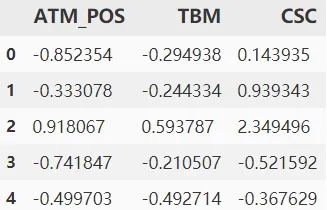3.1 K-Means 聚类的第一种方式

不进行变量分布的正太转换--用于寻找异常值

# 使用k-means聚类
## 1.1 k-means聚类的第一种方式：不进行变量分布的正态转换--用于寻找异常值

# 1、查看变量的偏度
var = ["ATM_POS","TBM","CSC"]  # var: variable-变量
skew_var = {}
for i in var:
skew_var[i]=abs(df[i].skew()) # .skew() 求该变量的偏度
skew=pd.Series(skew_var).sort_values(ascending=False)
skew

可以看出 TBM 这个变量的偏度已经超标，很可能会影响到后续的分类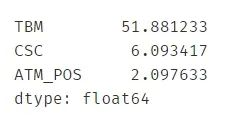进行k-means聚类

from sklearn.cluster import KMeans
kmeans = KMeans(n_clusters=3) # n_clusters=3 表示聚成3类

result = kmeans.fit(df)
result

与随机森林，决策树等算法一样，KMeans 函数中的参数众多，这里不具体解释了，可查阅官方文档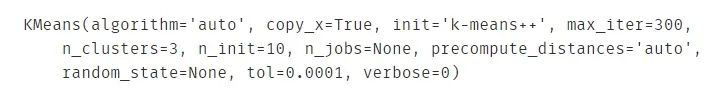.join() 表示横向拼接

# 对分类结果进行解读
model_data_l = df.join(pd.DataFrame(result.labels_))
# .labels_ 表示这一个数据点属于什么类
model_data_l = model_data_l.rename(columns={0: "clustor"})
model_data_l.sample(10)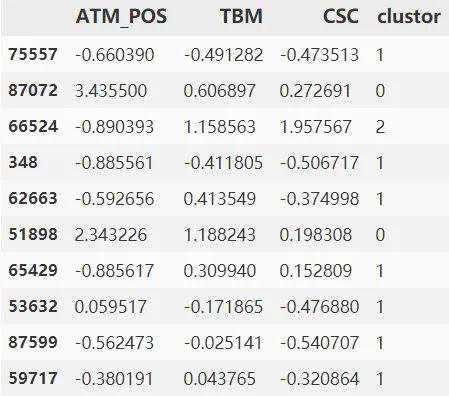绘制饼图呈现每一类的比例

# 饼图呈现
import matplotlib
get_ipython().magic('matplotlib inline')
model_data_l.clustor.value_counts().plot(kind = 'pie')
# 自然就能发现出现分类很不平均的现象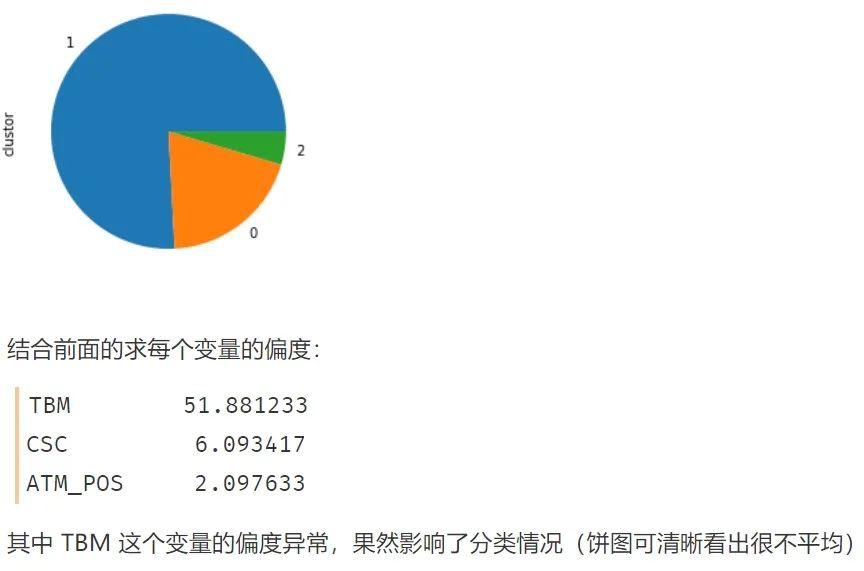3.2 k-means聚类的第二种方式

进行变量分布的正态转换--用于客户细分

# 进行变量分布的正态转换
import numpy as np
from sklearn import preprocessing
quantile_transformer = \
preprocessing.QuantileTransformer(output_distribution='normal',
random_state=0) # 正态转换

df_trans = quantile_transformer.fit_transform(df)
df_trans = pd.DataFrame(df_trans)
# 因为 .fit_transform 转换出来的数据类型为 Series，
## 所以用 pandas 给 DataFrame 化一下

df_trans = df_trans.rename(columns={0: "ATM_POS", 1: "TBM", 2: "CSC"})

转换的方式有很多种，每种都会涉及一些咋看起来比较晦涩的统计学公式,但请不要担心，每种代码其实都是比较固定的，这里使用 QT 转换（每种转换的原理和特点优劣等可参考网络资源）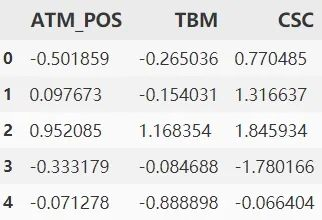检验一下偏度：发现几乎都为 0 了

var = ["ATM_POS","TBM","CSC"]
skew_var = {}

循环计算偏度：发现都差不多等于 0 了。

for i in var:
skew_var[i] = abs(df_trans[i].skew())
skew = pd.Series(skew_var).sort_values(ascending=False)
skew  # 字典显示更方便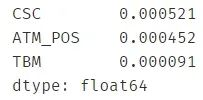重复的聚类步骤，代码可直接粘贴

kmeans = KMeans(n_clusters=4)  # 这次聚成 4 类
result = kmeans.fit(df_trans)

model_data_l = df_trans.join(pd.DataFrame(result.labels_))
model_data_l = model_data_l.rename(columns={0: "clustor"})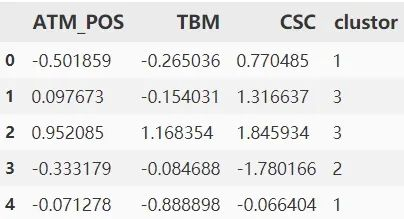再次使用饼图呈现结果，发现每类的比例开始平均了。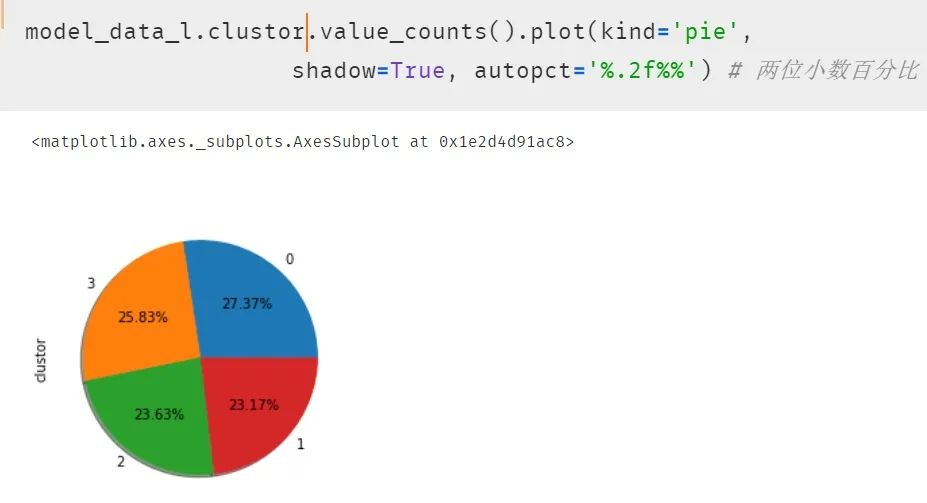3.3 结果分析

最后对结果进行分析如下表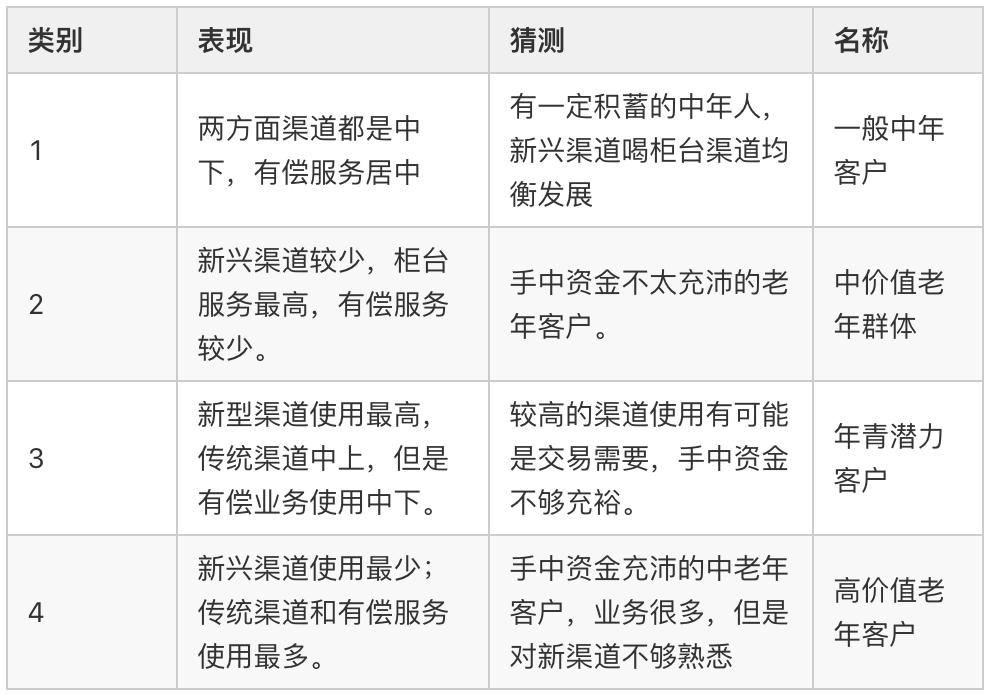小结

对于不同场景，我们的使用聚类的方法也有所不同：

• 一般场景下的聚类：「变量归一化  --> 分布转换 --> 主成分 --> 聚类」

• 发现异常境况的聚类：「变量归一化 --> 主成分 --> 聚类」

其实聚类模型对分析人员的业务修养要求较高，因为聚类结果好坏不是简单的看统计指标就可得出明确的答案。统计指标是在所有的变量都符合某个假设条件才能表现良好的，而实际建模中很少能达到那种状态；聚类的结果要做详细的描述性统计，甚至作抽样的客户访谈，以了解客户的真实情况，所以让业务人员满足客户管理的目标，是聚类的终极目标。

本系列源码可以在后台回复「数据分析实战」获取

—更多精彩文章，点击下方图片查看—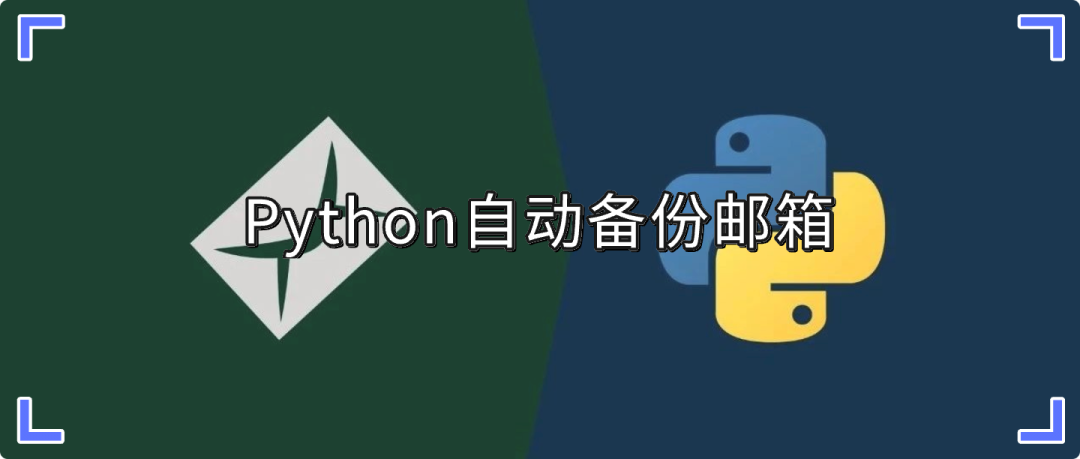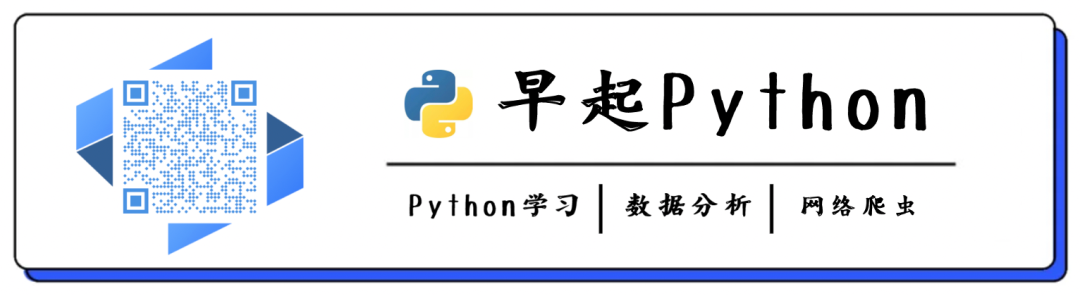展开全文weixin_41846769 2021-04-24 00:52:55
• 10 种 Python 聚类算法完整操作示例（附代码说明） 算法 大数据 数据挖掘 python 机器学习

来源 | 海豚数据科学实验室著作权归作者所有，本文仅作学术分享，若侵权，请联系后台删文处理聚类或聚类分析是无监督学习问题。它通常被用作数据分析技术，用于发现数据中的有趣模式，例如基于其行为...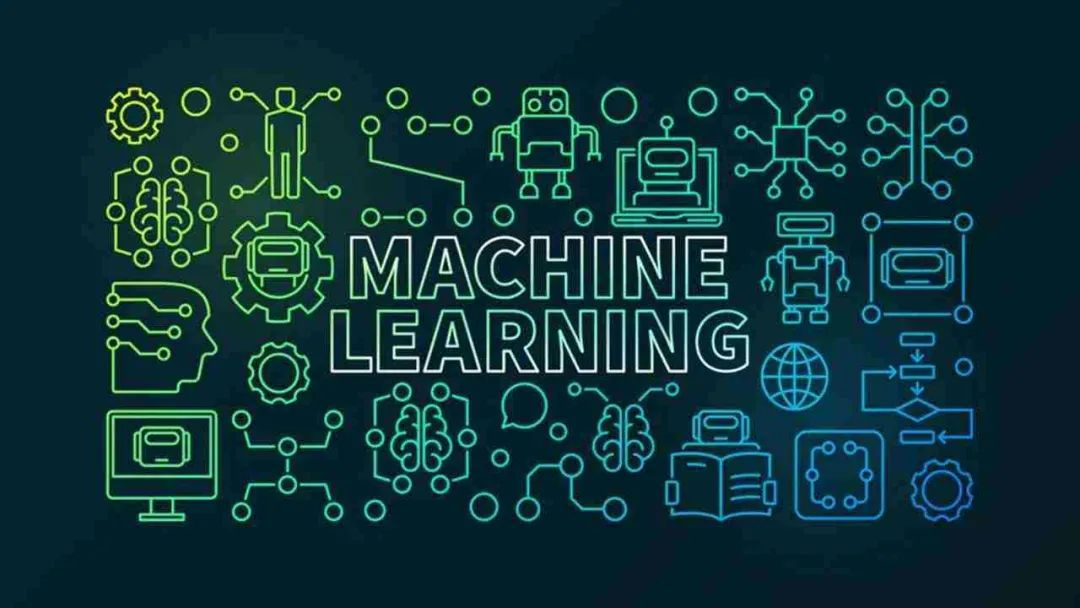来源 | 海豚数据科学实验室

著作权归作者所有，本文仅作学术分享，若侵权，请联系后台删文处理

聚类或聚类分析是无监督学习问题。它通常被用作数据分析技术，用于发现数据中的有趣模式，例如基于其行为的客户群。有许多聚类算法可供选择，对于所有情况，没有单一的最佳聚类算法。相反，最好探索一系列聚类算法以及每种算法的不同配置。在本教程中，你将发现如何在 python 中安装和使用顶级聚类算法。

完成本教程后，你将知道：

• 聚类是在输入数据的特征空间中查找自然组的无监督问题。

• 对于所有数据集，有许多不同的聚类算法和单一的最佳方法。

• 在 scikit-learn 机器学习库的 Python 中如何实现、适配和使用顶级聚类算法。

让我们开始吧。

教程概述

本教程分为三部分：

1. 聚类

2. 聚类算法

3. 聚类算法示例

• 库安装

• 聚类数据集

• 亲和力传播

• 聚合聚类

• BIRCH

• DBSCAN

• K-均值

• Mini-Batch K-均值

• Mean Shift

• OPTICS

• 光谱聚类

• 高斯混合模型

一、聚类

聚类分析，即聚类，是一项无监督的机器学习任务。它包括自动发现数据中的自然分组。与监督学习（类似预测建模）不同，聚类算法只解释输入数据，并在特征空间中找到自然组或群集。

聚类技术适用于没有要预测的类，而是将实例划分为自然组的情况。
—源自：《数据挖掘页：实用机器学习工具和技术》2016年。

群集通常是特征空间中的密度区域，其中来自域的示例（观测或数据行）比其他群集更接近群集。群集可以具有作为样本或点特征空间的中心(质心)，并且可以具有边界或范围。

这些群集可能反映出在从中绘制实例的域中工作的某种机制，这种机制使某些实例彼此具有比它们与其余实例更强的相似性。
—源自：《数据挖掘页：实用机器学习工具和技术》2016年。

聚类可以作为数据分析活动提供帮助，以便了解更多关于问题域的信息，即所谓的模式发现或知识发现。例如：

• 该进化树可以被认为是人工聚类分析的结果；

• 将正常数据与异常值或异常分开可能会被认为是聚类问题；

• 根据自然行为将集群分开是一个集群问题，称为市场细分。

聚类还可用作特征工程的类型，其中现有的和新的示例可被映射并标记为属于数据中所标识的群集之一。虽然确实存在许多特定于群集的定量措施，但是对所识别的群集的评估是主观的，并且可能需要领域专家。通常，聚类算法在人工合成数据集上与预先定义的群集进行学术比较，预计算法会发现这些群集。

聚类是一种无监督学习技术，因此很难评估任何给定方法的输出质量。
—源自：《机器学习页：概率观点》2012。

二、聚类算法

有许多类型的聚类算法。许多算法在特征空间中的示例之间使用相似度或距离度量，以发现密集的观测区域。因此，在使用聚类算法之前，扩展数据通常是良好的实践。

聚类分析的所有目标的核心是被群集的各个对象之间的相似程度（或不同程度）的概念。聚类方法尝试根据提供给对象的相似性定义对对象进行分组。
—源自：《统计学习的要素：数据挖掘、推理和预测》，2016年

一些聚类算法要求您指定或猜测数据中要发现的群集的数量，而另一些算法要求指定观测之间的最小距离，其中示例可以被视为“关闭”或“连接”。因此，聚类分析是一个迭代过程，在该过程中，对所识别的群集的主观评估被反馈回算法配置的改变中，直到达到期望的或适当的结果。scikit-learn 库提供了一套不同的聚类算法供选择。下面列出了10种比较流行的算法：

1. 亲和力传播

2. 聚合聚类

3. BIRCH

4. DBSCAN

5. K-均值

6. Mini-Batch K-均值

7. Mean Shift

8. OPTICS

9. 光谱聚类

10. 高斯混合

每个算法都提供了一种不同的方法来应对数据中发现自然组的挑战。没有最好的聚类算法，也没有简单的方法来找到最好的算法为您的数据没有使用控制实验。在本教程中，我们将回顾如何使用来自 scikit-learn 库的这10个流行的聚类算法中的每一个。这些示例将为您复制粘贴示例并在自己的数据上测试方法提供基础。我们不会深入研究算法如何工作的理论，也不会直接比较它们。让我们深入研究一下。

三. 聚类算法示例

在本节中，我们将回顾如何在 scikit-learn 中使用10个流行的聚类算法。这包括一个拟合模型的例子和可视化结果的例子。这些示例用于将粘贴复制到您自己的项目中，并将方法应用于您自己的数据。

1.库安装

首先，让我们安装库。不要跳过此步骤，因为你需要确保安装了最新版本。你可以使用 pip Python 安装程序安装 scikit-learn 存储库，如下所示：

sudo pip install scikit-learn

接下来，让我们确认已经安装了库，并且您正在使用一个现代版本。运行以下脚本以输出库版本号。

# 检查 scikit-learn 版本
import sklearn
print(sklearn.__version__)

运行该示例时，您应该看到以下版本号或更高版本。

0.22.1

2.聚类数据集

我们将使用 make _ classification ()函数创建一个测试二分类数据集。数据集将有1000个示例，每个类有两个输入要素和一个群集。这些群集在两个维度上是可见的，因此我们可以用散点图绘制数据，并通过指定的群集对图中的点进行颜色绘制。

这将有助于了解，至少在测试问题上，群集的识别能力如何。该测试问题中的群集基于多变量高斯，并非所有聚类算法都能有效地识别这些类型的群集。因此，本教程中的结果不应用作比较一般方法的基础。下面列出了创建和汇总合成聚类数据集的示例。

# 综合分类数据集
from numpy import where
from sklearn.datasets import make_classification
from matplotlib import pyplot
# 定义数据集
X, y = make_classification(n_samples=1000, n_features=2, n_informative=2, n_redundant=0, n_clusters_per_class=1, random_state=4)
# 为每个类的样本创建散点图
for class_value in range(2):
# 获取此类的示例的行索引
row_ix = where(y == class_value)
# 创建这些样本的散布
pyplot.scatter(X[row_ix, 0], X[row_ix, 1])
# 绘制散点图
pyplot.show()

运行该示例将创建合成的聚类数据集，然后创建输入数据的散点图，其中点由类标签（理想化的群集）着色。我们可以清楚地看到两个不同的数据组在两个维度，并希望一个自动的聚类算法可以检测这些分组。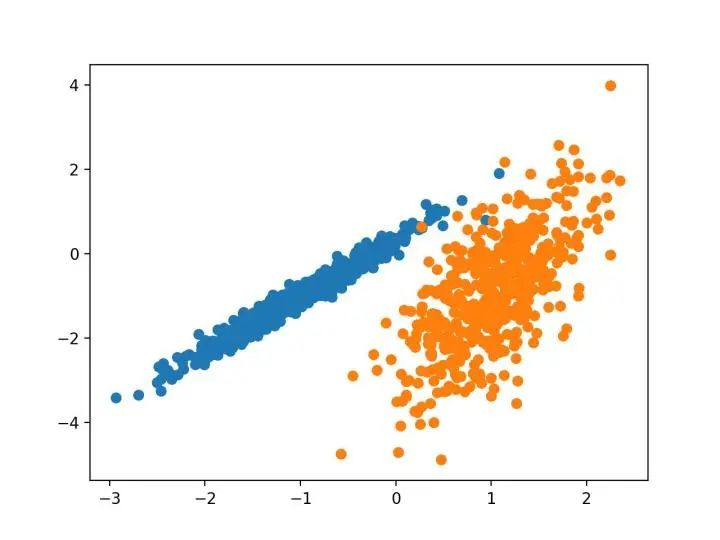已知聚类着色点的合成聚类数据集的散点图

接下来，我们可以开始查看应用于此数据集的聚类算法的示例。我已经做了一些最小的尝试来调整每个方法到数据集。

3.亲和力传播

亲和力传播包括找到一组最能概括数据的范例。

我们设计了一种名为“亲和传播”的方法，它作为两对数据点之间相似度的输入度量。在数据点之间交换实值消息，直到一组高质量的范例和相应的群集逐渐出现
—源自：《通过在数据点之间传递消息》2007。

它是通过 AffinityPropagation 类实现的，要调整的主要配置是将“ 阻尼 ”设置为0.5到1，甚至可能是“首选项”。

下面列出了完整的示例。

# 亲和力传播聚类
from numpy import unique
from numpy import where
from sklearn.datasets import make_classification
from sklearn.cluster import AffinityPropagation
from matplotlib import pyplot
# 定义数据集
X, _ = make_classification(n_samples=1000, n_features=2, n_informative=2, n_redundant=0, n_clusters_per_class=1, random_state=4)
# 定义模型
model = AffinityPropagation(damping=0.9)
# 匹配模型
model.fit(X)
# 为每个示例分配一个集群
yhat = model.predict(X)
# 检索唯一群集
clusters = unique(yhat)
# 为每个群集的样本创建散点图
for cluster in clusters:
# 获取此群集的示例的行索引
row_ix = where(yhat == cluster)
# 创建这些样本的散布
pyplot.scatter(X[row_ix, 0], X[row_ix, 1])
# 绘制散点图
pyplot.show()

运行该示例符合训练数据集上的模型，并预测数据集中每个示例的群集。然后创建一个散点图，并由其指定的群集着色。在这种情况下，我无法取得良好的结果。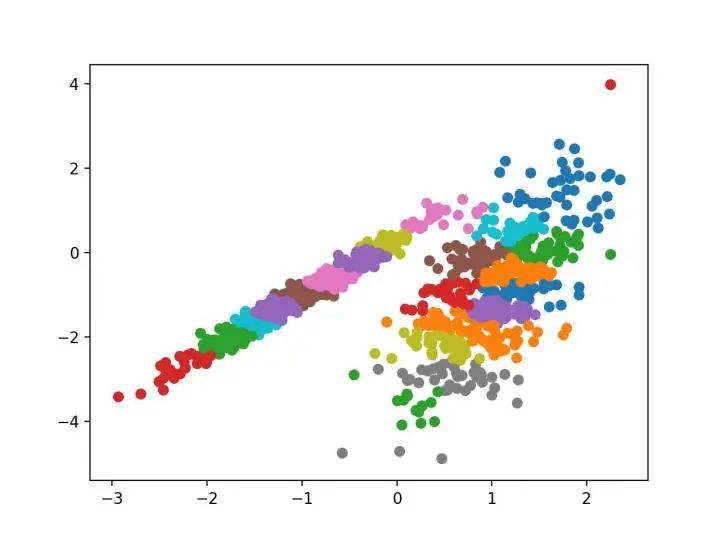数据集的散点图，具有使用亲和力传播识别的聚类

4.聚合聚类

聚合聚类涉及合并示例，直到达到所需的群集数量为止。它是层次聚类方法的更广泛类的一部分，通过 AgglomerationClustering 类实现的，主要配置是“ n _ clusters ”集，这是对数据中的群集数量的估计，例如2。下面列出了完整的示例。

# 聚合聚类
from numpy import unique
from numpy import where
from sklearn.datasets import make_classification
from sklearn.cluster import AgglomerativeClustering
from matplotlib import pyplot
# 定义数据集
X, _ = make_classification(n_samples=1000, n_features=2, n_informative=2, n_redundant=0, n_clusters_per_class=1, random_state=4)
# 定义模型
model = AgglomerativeClustering(n_clusters=2)
# 模型拟合与聚类预测
yhat = model.fit_predict(X)
# 检索唯一群集
clusters = unique(yhat)
# 为每个群集的样本创建散点图
for cluster in clusters:
# 获取此群集的示例的行索引
row_ix = where(yhat == cluster)
# 创建这些样本的散布
pyplot.scatter(X[row_ix, 0], X[row_ix, 1])
# 绘制散点图
pyplot.show()

运行该示例符合训练数据集上的模型，并预测数据集中每个示例的群集。然后创建一个散点图，并由其指定的群集着色。在这种情况下，可以找到一个合理的分组。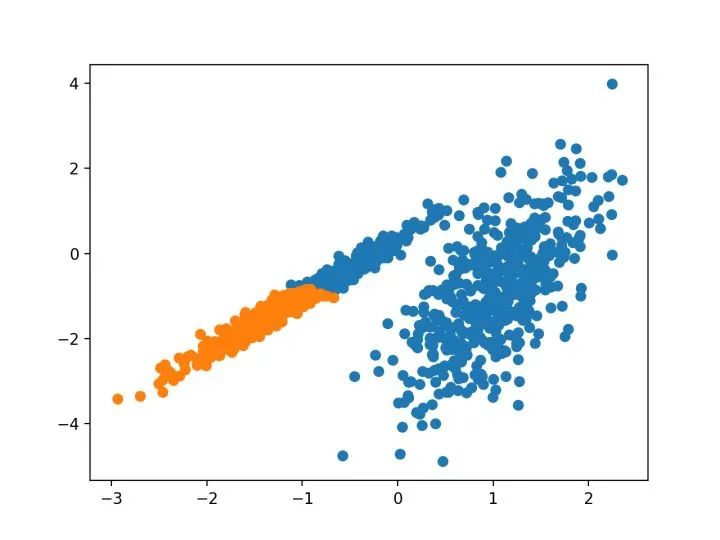使用聚集聚类识别出具有聚类的数据集的散点图

5.BIRCH

BIRCH 聚类（ BIRCH 是平衡迭代减少的缩写，聚类使用层次结构)包括构造一个树状结构，从中提取聚类质心。

BIRCH 递增地和动态地群集传入的多维度量数据点，以尝试利用可用资源（即可用内存和时间约束）产生最佳质量的聚类。
—源自：《 BIRCH ：1996年大型数据库的高效数据聚类方法》

它是通过 Birch 类实现的，主要配置是“ threshold ”和“ n _ clusters ”超参数，后者提供了群集数量的估计。下面列出了完整的示例。

# birch聚类
from numpy import unique
from numpy import where
from sklearn.datasets import make_classification
from sklearn.cluster import Birch
from matplotlib import pyplot
# 定义数据集
X, _ = make_classification(n_samples=1000, n_features=2, n_informative=2, n_redundant=0, n_clusters_per_class=1, random_state=4)
# 定义模型
model = Birch(threshold=0.01, n_clusters=2)
# 适配模型
model.fit(X)
# 为每个示例分配一个集群
yhat = model.predict(X)
# 检索唯一群集
clusters = unique(yhat)
# 为每个群集的样本创建散点图
for cluster in clusters:
# 获取此群集的示例的行索引
row_ix = where(yhat == cluster)
# 创建这些样本的散布
pyplot.scatter(X[row_ix, 0], X[row_ix, 1])
# 绘制散点图
pyplot.show()

运行该示例符合训练数据集上的模型，并预测数据集中每个示例的群集。然后创建一个散点图，并由其指定的群集着色。在这种情况下，可以找到一个很好的分组。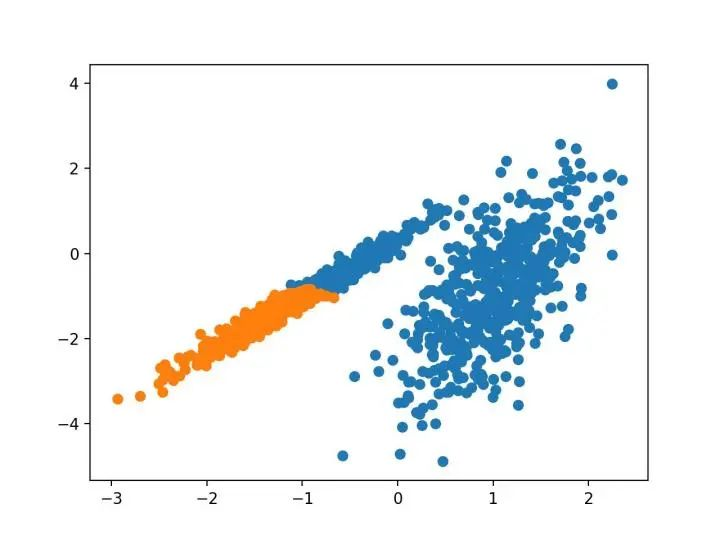使用BIRCH聚类确定具有聚类的数据集的散点图

6.DBSCAN

DBSCAN 聚类（其中 DBSCAN 是基于密度的空间聚类的噪声应用程序）涉及在域中寻找高密度区域，并将其周围的特征空间区域扩展为群集。

…我们提出了新的聚类算法 DBSCAN 依赖于基于密度的概念的集群设计，以发现任意形状的集群。DBSCAN 只需要一个输入参数，并支持用户为其确定适当的值
-源自：《基于密度的噪声大空间数据库聚类发现算法》，1996

它是通过 DBSCAN 类实现的，主要配置是“ eps ”和“ min _ samples ”超参数。

下面列出了完整的示例。

# dbscan 聚类
from numpy import unique
from numpy import where
from sklearn.datasets import make_classification
from sklearn.cluster import DBSCAN
from matplotlib import pyplot
# 定义数据集
X, _ = make_classification(n_samples=1000, n_features=2, n_informative=2, n_redundant=0, n_clusters_per_class=1, random_state=4)
# 定义模型
model = DBSCAN(eps=0.30, min_samples=9)
# 模型拟合与聚类预测
yhat = model.fit_predict(X)
# 检索唯一群集
clusters = unique(yhat)
# 为每个群集的样本创建散点图
for cluster in clusters:
# 获取此群集的示例的行索引
row_ix = where(yhat == cluster)
# 创建这些样本的散布
pyplot.scatter(X[row_ix, 0], X[row_ix, 1])
# 绘制散点图
pyplot.show()

运行该示例符合训练数据集上的模型，并预测数据集中每个示例的群集。然后创建一个散点图，并由其指定的群集着色。在这种情况下，尽管需要更多的调整，但是找到了合理的分组。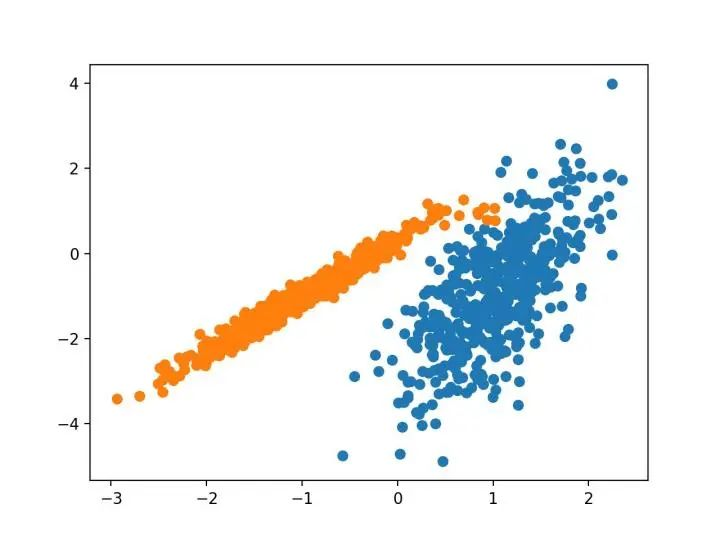使用DBSCAN集群识别出具有集群的数据集的散点图

7.K均值

K-均值聚类可以是最常见的聚类算法，并涉及向群集分配示例，以尽量减少每个群集内的方差。

本文的主要目的是描述一种基于样本将 N 维种群划分为 k 个集合的过程。这个叫做“ K-均值”的过程似乎给出了在类内方差意义上相当有效的分区。
-源自：《关于多元观测的分类和分析的一些方法》1967年。

它是通过 K-均值类实现的，要优化的主要配置是“ n _ clusters ”超参数设置为数据中估计的群集数量。下面列出了完整的示例。

# k-means 聚类
from numpy import unique
from numpy import where
from sklearn.datasets import make_classification
from sklearn.cluster import KMeans
from matplotlib import pyplot
# 定义数据集
X, _ = make_classification(n_samples=1000, n_features=2, n_informative=2, n_redundant=0, n_clusters_per_class=1, random_state=4)
# 定义模型
model = KMeans(n_clusters=2)
# 模型拟合
model.fit(X)
# 为每个示例分配一个集群
yhat = model.predict(X)
# 检索唯一群集
clusters = unique(yhat)
# 为每个群集的样本创建散点图
for cluster in clusters:
# 获取此群集的示例的行索引
row_ix = where(yhat == cluster)
# 创建这些样本的散布
pyplot.scatter(X[row_ix, 0], X[row_ix, 1])
# 绘制散点图
pyplot.show()

运行该示例符合训练数据集上的模型，并预测数据集中每个示例的群集。然后创建一个散点图，并由其指定的群集着色。在这种情况下，可以找到一个合理的分组，尽管每个维度中的不等等方差使得该方法不太适合该数据集。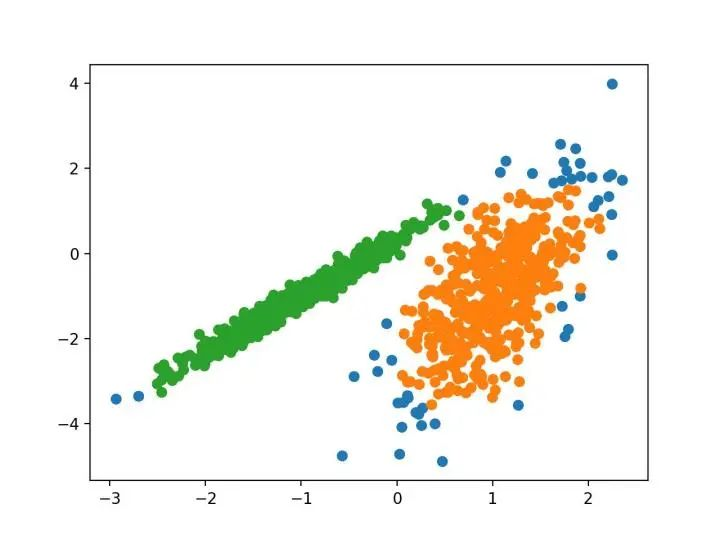使用K均值聚类识别出具有聚类的数据集的散点图

8.Mini-Batch K-均值

Mini-Batch K-均值是 K-均值的修改版本，它使用小批量的样本而不是整个数据集对群集质心进行更新，这可以使大数据集的更新速度更快，并且可能对统计噪声更健壮。

...我们建议使用 k-均值聚类的迷你批量优化。与经典批处理算法相比，这降低了计算成本的数量级，同时提供了比在线随机梯度下降更好的解决方案。
—源自：《Web-Scale K-均值聚类》2010

它是通过 MiniBatchKMeans 类实现的，要优化的主配置是“ n _ clusters ”超参数，设置为数据中估计的群集数量。下面列出了完整的示例。

# mini-batch k均值聚类
from numpy import unique
from numpy import where
from sklearn.datasets import make_classification
from sklearn.cluster import MiniBatchKMeans
from matplotlib import pyplot
# 定义数据集
X, _ = make_classification(n_samples=1000, n_features=2, n_informative=2, n_redundant=0, n_clusters_per_class=1, random_state=4)
# 定义模型
model = MiniBatchKMeans(n_clusters=2)
# 模型拟合
model.fit(X)
# 为每个示例分配一个集群
yhat = model.predict(X)
# 检索唯一群集
clusters = unique(yhat)
# 为每个群集的样本创建散点图
for cluster in clusters:
# 获取此群集的示例的行索引
row_ix = where(yhat == cluster)
# 创建这些样本的散布
pyplot.scatter(X[row_ix, 0], X[row_ix, 1])
# 绘制散点图
pyplot.show()

运行该示例符合训练数据集上的模型，并预测数据集中每个示例的群集。然后创建一个散点图，并由其指定的群集着色。在这种情况下，会找到与标准 K-均值算法相当的结果。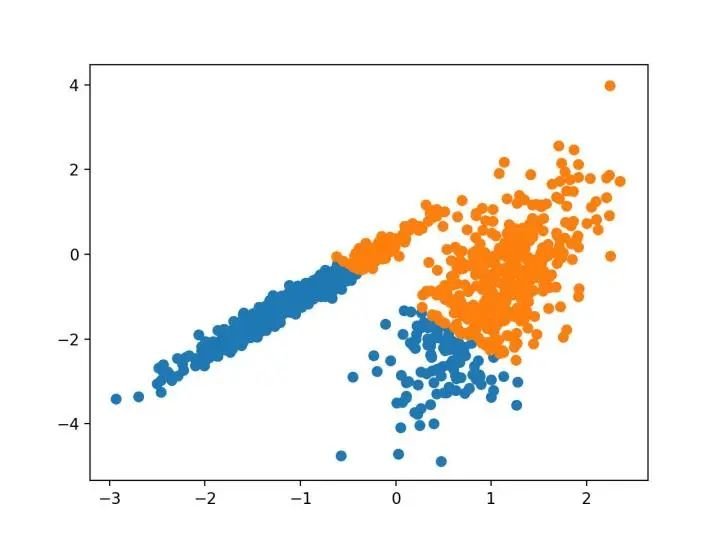带有最小批次K均值聚类的聚类数据集的散点图

9.均值漂移聚类

均值漂移聚类涉及到根据特征空间中的实例密度来寻找和调整质心。

对离散数据证明了递推平均移位程序收敛到最接近驻点的基础密度函数，从而证明了它在检测密度模式中的应用。
—源自：《Mean Shift ：面向特征空间分析的稳健方法》，2002

它是通过 MeanShift 类实现的，主要配置是“带宽”超参数。下面列出了完整的示例。

# 均值漂移聚类
from numpy import unique
from numpy import where
from sklearn.datasets import make_classification
from sklearn.cluster import MeanShift
from matplotlib import pyplot
# 定义数据集
X, _ = make_classification(n_samples=1000, n_features=2, n_informative=2, n_redundant=0, n_clusters_per_class=1, random_state=4)
# 定义模型
model = MeanShift()
# 模型拟合与聚类预测
yhat = model.fit_predict(X)
# 检索唯一群集
clusters = unique(yhat)
# 为每个群集的样本创建散点图
for cluster in clusters:
# 获取此群集的示例的行索引
row_ix = where(yhat == cluster)
# 创建这些样本的散布
pyplot.scatter(X[row_ix, 0], X[row_ix, 1])
# 绘制散点图
pyplot.show()

运行该示例符合训练数据集上的模型，并预测数据集中每个示例的群集。然后创建一个散点图，并由其指定的群集着色。在这种情况下，可以在数据中找到一组合理的群集。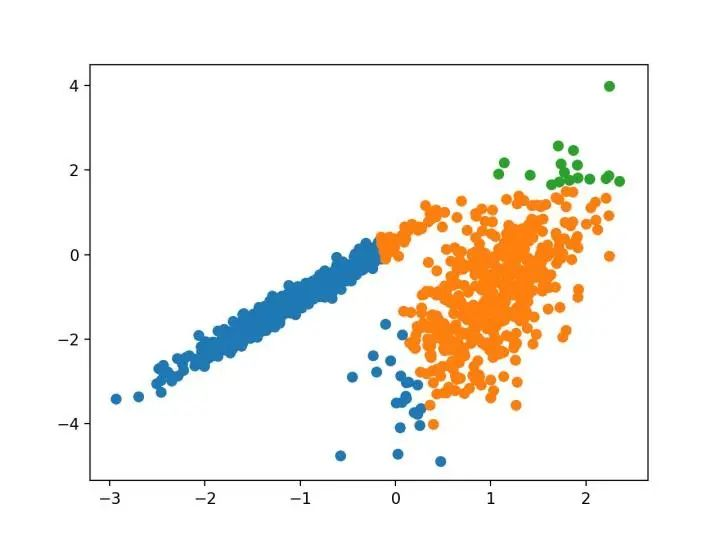具有均值漂移聚类的聚类数据集散点图

10.OPTICS

OPTICS 聚类（ OPTICS 短于订购点数以标识聚类结构）是上述 DBSCAN 的修改版本。

我们为聚类分析引入了一种新的算法，它不会显式地生成一个数据集的聚类；而是创建表示其基于密度的聚类结构的数据库的增强排序。此群集排序包含相当于密度聚类的信息，该信息对应于范围广泛的参数设置。
—源自：《OPTICS ：排序点以标识聚类结构》，1999

它是通过 OPTICS 类实现的，主要配置是“ eps ”和“ min _ samples ”超参数。下面列出了完整的示例。

# optics聚类
from numpy import unique
from numpy import where
from sklearn.datasets import make_classification
from sklearn.cluster import OPTICS
from matplotlib import pyplot
# 定义数据集
X, _ = make_classification(n_samples=1000, n_features=2, n_informative=2, n_redundant=0, n_clusters_per_class=1, random_state=4)
# 定义模型
model = OPTICS(eps=0.8, min_samples=10)
# 模型拟合与聚类预测
yhat = model.fit_predict(X)
# 检索唯一群集
clusters = unique(yhat)
# 为每个群集的样本创建散点图
for cluster in clusters:
# 获取此群集的示例的行索引
row_ix = where(yhat == cluster)
# 创建这些样本的散布
pyplot.scatter(X[row_ix, 0], X[row_ix, 1])
# 绘制散点图
pyplot.show()

运行该示例符合训练数据集上的模型，并预测数据集中每个示例的群集。然后创建一个散点图，并由其指定的群集着色。在这种情况下，我无法在此数据集上获得合理的结果。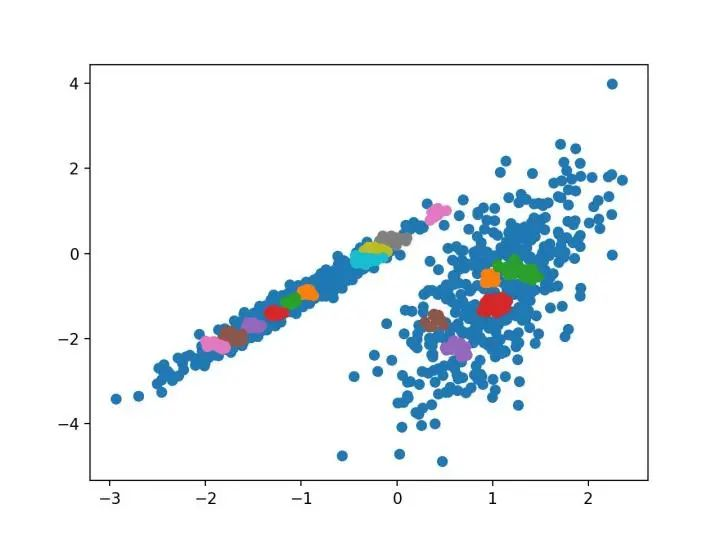使用OPTICS聚类确定具有聚类的数据集的散点图

11.光谱聚类

光谱聚类是一类通用的聚类方法，取自线性线性代数。

最近在许多领域出现的一个有希望的替代方案是使用聚类的光谱方法。这里，使用从点之间的距离导出的矩阵的顶部特征向量。
—源自：《关于光谱聚类：分析和算法》，2002年

它是通过 Spectral 聚类类实现的，而主要的 Spectral 聚类是一个由聚类方法组成的通用类，取自线性线性代数。要优化的是“ n _ clusters ”超参数，用于指定数据中的估计群集数量。下面列出了完整的示例。

# spectral clustering
from numpy import unique
from numpy import where
from sklearn.datasets import make_classification
from sklearn.cluster import SpectralClustering
from matplotlib import pyplot
# 定义数据集
X, _ = make_classification(n_samples=1000, n_features=2, n_informative=2, n_redundant=0, n_clusters_per_class=1, random_state=4)
# 定义模型
model = SpectralClustering(n_clusters=2)
# 模型拟合与聚类预测
yhat = model.fit_predict(X)
# 检索唯一群集
clusters = unique(yhat)
# 为每个群集的样本创建散点图
for cluster in clusters:
# 获取此群集的示例的行索引
row_ix = where(yhat == cluster)
# 创建这些样本的散布
pyplot.scatter(X[row_ix, 0], X[row_ix, 1])
# 绘制散点图
pyplot.show()

运行该示例符合训练数据集上的模型，并预测数据集中每个示例的群集。然后创建一个散点图，并由其指定的群集着色。

在这种情况下，找到了合理的集群。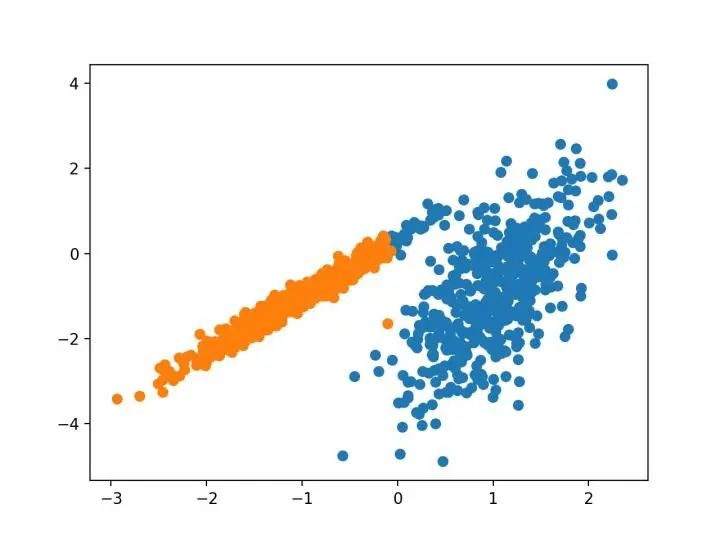使用光谱聚类聚类识别出具有聚类的数据集的散点图

12.高斯混合模型

高斯混合模型总结了一个多变量概率密度函数，顾名思义就是混合了高斯概率分布。它是通过 Gaussian Mixture 类实现的，要优化的主要配置是“ n _ clusters ”超参数，用于指定数据中估计的群集数量。下面列出了完整的示例。

# 高斯混合模型
from numpy import unique
from numpy import where
from sklearn.datasets import make_classification
from sklearn.mixture import GaussianMixture
from matplotlib import pyplot
# 定义数据集
X, _ = make_classification(n_samples=1000, n_features=2, n_informative=2, n_redundant=0, n_clusters_per_class=1, random_state=4)
# 定义模型
model = GaussianMixture(n_components=2)
# 模型拟合
model.fit(X)
# 为每个示例分配一个集群
yhat = model.predict(X)
# 检索唯一群集
clusters = unique(yhat)
# 为每个群集的样本创建散点图
for cluster in clusters:
# 获取此群集的示例的行索引
row_ix = where(yhat == cluster)
# 创建这些样本的散布
pyplot.scatter(X[row_ix, 0], X[row_ix, 1])
# 绘制散点图
pyplot.show()

运行该示例符合训练数据集上的模型，并预测数据集中每个示例的群集。然后创建一个散点图，并由其指定的群集着色。在这种情况下，我们可以看到群集被完美地识别。这并不奇怪，因为数据集是作为 Gaussian 的混合生成的。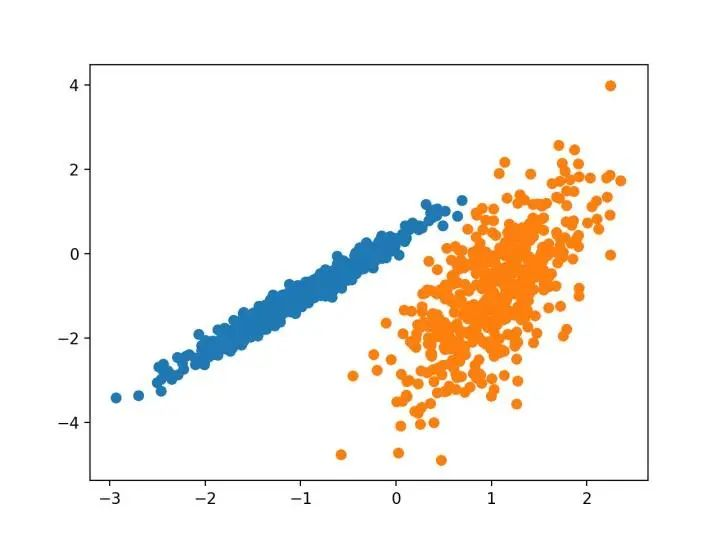使用高斯混合聚类识别出具有聚类的数据集的散点图

四.总结

在本教程中，您发现了如何在 python 中安装和使用顶级聚类算法。具体来说，你学到了：

• 聚类是在特征空间输入数据中发现自然组的无监督问题。

• 有许多不同的聚类算法，对于所有数据集没有单一的最佳方法。

• 在 scikit-learn 机器学习库的 Python 中如何实现、适合和使用顶级聚类算法。

展开全文lsxxx2011 2021-03-17 00:23:38
• python进行聚类分析：鸢尾花（iris）代码 python 聚类 算法

python进行聚类分析：鸢尾花（iris）代码基本信息代码实现基础函数KemansMiniBatchKMeansMeanShiftAgglomerativeClusteringDBSCANBirchSpectralClusteringAffinityPropagation总结 基本信息 在无监督学习中，数据不...

基本信息

在无监督学习中，数据不带任何标签。能找出数据内在分类规则，并分成独立的点集（蔟），算法称为聚类算法。
本文主要利用经典的鸢尾花（iris）来实现python的简单的聚类分析。

代码实现

基础函数

# 导入库
import matplotlib.pyplot as plt
import numpy as np
from sklearn import datasets
import time
from sklearn import metrics
import matplotlib as mpl

## 导入数据
X = iris.data[:, :4]  # #表示我们取特征空间中的4个维度
Y = iris.target
print(X.shape)

## 定义基本函数
def get_HT(X,Y,label_pred,name='k_means'):
mpl.rcParams['font.sans-serif'] = ['SimHei']
mpl.rcParams['axes.unicode_minus'] = False

x0 = X[label_pred == 0]
x1 = X[label_pred == 1]
x2 = X[label_pred == 2]
plt.scatter(x0[:, 0], x0[:, 1], c="red", marker='o', label='簇1')
plt.scatter(x1[:, 0], x1[:, 1], c="green", marker='*', label='簇2')
plt.scatter(x2[:, 0], x2[:, 1], c="blue", marker='+', label='簇3')
plt.xlabel('sepal length')
plt.ylabel('sepal width')
plt.legend(loc=2)
plt.title(name + "聚类结果")
plt.show()

score_funcs = [
metrics.v_measure_score,        #均一性与完整性的加权平均
metrics.mutual_info_score,      #互信息
]

sum = 0
for score_func in score_funcs:
km_scores = score_func(Y, label_pred)
sum += km_scores
print(name + "算法:%s评估函数计算结果值:%.5f" % (score_func.__name__, km_scores))
print('平均分数为：%.2f'%(sum/4))

Kemans

#Kmeans算法
from sklearn.cluster import KMeans
estimator = KMeans(n_clusters=3,max_iter = 200)  # 构造聚类器
estimator.fit(X)  # 聚类

label_pred = estimator.labels_  # 获取聚类标签
get_HT(X,Y = Y,label_pred = label_pred,name = 'KMeans')MiniBatchKMeans

from sklearn.cluster import MiniBatchKMeans
estimator = MiniBatchKMeans(n_clusters=3,init='k-means++',max_no_improvement = 10,
batch_size = 30,random_state=28)  # 构造聚类器
estimator.fit(X)  # 聚类
label_pred = estimator.labels_  # 获取聚类标签
get_HT(X,Y = Y,label_pred = label_pred,name = 'MiniBatchKMeans')MeanShift

from sklearn.cluster import MeanShift
#  均值漂移,是一种核密度估计算法，它将每个点移动到密度函数的局部极大值点处
## Mean Shift算法,一般是指一个迭代的步骤,即先算出当前点的偏移均值,移动该点到其偏移均值,然后以此为新的起始点,继续移动,
## 直到满足一定的条件结束
ms = MeanShift(bandwidth=0.8,seeds=None,bin_seeding=False,min_bin_freq=1,cluster_all=True,n_jobs=1)
res = ms.fit(X)
label_pred = res.labels_  # 获取聚类标签

get_HT(X,Y = Y,label_pred = label_pred,name = 'MeanShift')AgglomerativeClustering

from sklearn.cluster import AgglomerativeClustering
res = clustering.fit(X)
label_pred = res.labels_  # 获取聚类标签

get_HT(X,Y = Y,label_pred = label_pred,name = 'AgglomerativeClustering')DBSCAN

from sklearn.cluster import DBSCAN
dbscan = DBSCAN(eps=0.4, min_samples=9)
dbscan.fit(X)
label_pred = dbscan.labels_

get_HT(X,Y = Y,label_pred = label_pred,name = 'DBSCAN')Birch

from sklearn.cluster import Birch
birch = Birch(threshold=0.5,branching_factor=50,n_clusters=3,compute_labels=True,copy=True)
#threshold：subcluster的半径，如果某一样本点的加入，使subcluster半径超过这个值，则该样本会被分到其它的subcluster；默认值是0.5.如果样本的方差较大，则一般需要增大这个默认值。
#branching_factor：每个node拥有的CF个数，如果新加入的CF使node中的CF数超过设定值，则将该Node一分为二；默认是50。如果样本量非常大，比如大于10万，则一般需要增大这个默认值。
#n_clusters：想要得到的cluster数量；如果n_cluster=None，则输出叶子结点中所有CF；如果n_cluster= int，则将叶子节点中的CF再次进行聚类；如果n_cluster=instance of sklearn.cluster.model，则将叶子节点中的CF看作new data，利用该model进行聚类；
#copy=False：将overwritten data；
#compute_labels=True：布尔值，表示是否标示类别输出，默认是True。一般使用默认值挺好，这样可以看到聚类效果。
birch.fit(X)
label_pred = birch.labels_

get_HT(X,Y = Y,label_pred = label_pred,name = 'Birch')SpectralClustering

from sklearn.cluster import SpectralClustering
sc = SpectralClustering(n_clusters=8,eigen_solver=None,random_state=None,n_init=10,gamma=1.0,
affinity='rbf',n_neighbors=10,eigen_tol=0.0,assign_labels='kmeans',degree=3,coef0=1,kernel_params=None,n_jobs=1)
#n_clusters：聚类的个数；
#eigen_solver：使用的特征值分解策略；
#gamma：计算相似矩阵时，如果使用核函数，该核函数的系数；
#affinity：计算相似度时使用的距离公式；
#n_neighbors：利用k近邻方法构建邻接矩阵时，所使用的k近邻；
#eigen_tol：使用arpack eigen_solver时拉普拉斯矩阵特征分解的停止准则.
#assign_labels：通过求解“拉普拉斯矩阵特征值”，获得指示向量矩阵（类似于降维后的特征），在指示向量矩阵的基础上，对矩阵各行，运用assign_labels进行聚类
sc.fit(X)
label_pred = sc.labels_

get_HT(X,Y = Y,label_pred = label_pred,name = 'SpectralClustering')AffinityPropagation

from sklearn.cluster import AffinityPropagation
ap = AffinityPropagation(damping=0.9,max_iter=200,convergence_iter=15,copy=True,preference=None,affinity='euclidean',verbose=False)
#damping：减振因子，用来避免在iteration中r,a的来回震荡；
#convergence_iter：在estimated cluster数量不变后，还要进行的iteration次数；
#affinity：用来估计样本之间亲密度的函数：{precomputed,euclidean}，{事先定义，欧几里得}
ap.fit(X)
label_pred = ap.labels_

get_HT(X,Y = Y,label_pred = label_pred,name = 'AffinityPropagation')总结

更多聚类算法，可参考官方文档：https://scikit-learn.org/stable/modules/clustering.html#k-means。

作者简介
作者：榆尚天
简介：一个数据分析的爱好者，立志成为一名数据界的幽灵。
微信平台：数据幽灵期待与各位同道的交流，欢迎关注~~~
————————————————————————————
作者：榆尚天
出处：https://blog.csdn.net/weixin_42926612/article/details/107982065
说明：本文版权归作者所有，欢迎转载，但未经作者同意必须保留此段声明，且在文章页面明显位置给出原文连接。

展开全文weixin_42926612 2020-08-13 16:16:19
• 模糊聚类python可执行完整代码 模糊聚类

6KB qq_28266311 2019-03-15 20:54:24
• Python实现聚类分析并可视化 import matplotlib.pyplot as plt import numpy as np import pandas as pd from sklearn.cluster import KMeans from sklearn import datasets from pylab import * mpl.rcParams['font...

Python实现聚类分析并可视化

import matplotlib.pyplot as plt
import numpy as np
import pandas as pd
from sklearn.cluster import KMeans
from sklearn import datasets
from pylab import *
mpl.rcParams['font.sans-serif'] = ['SimHei']

data1=data.values
X = data1[:, 1:3]  # #表示我们取特征空间中的4个维度
#X = iris.data[:, :4]  # #表示我们取特征空间中的4个维度
print(X.shape)
print(X)
plt.figure(figsize=(12,8))
# 绘制数据分布图
plt.scatter(X[:, 0], X[:, 1], c="red", marker='o', label='see')
plt.xlabel('sepal length')
plt.ylabel('sepal width')
plt.legend(loc=2)
plt.show()

estimator = KMeans(n_clusters=4)  # 构造聚类器
estimator.fit(X)  # 聚类
label_pred = estimator.labels_  # 获取聚类标签
# 绘制k-means结果
x0 = X[label_pred == 0]
x1 = X[label_pred == 1]
x2 = X[label_pred == 2]
x3 = X[label_pred == 3]
plt.figure(figsize=(14,8))
plt.scatter(x0[:, 0], x0[:, 1], c='red', marker='o', label='佛山')
plt.scatter(x1[:, 0], x1[:, 1], c='green', marker='*', label='广州')
plt.scatter(x2[:, 0], x2[:, 1], c='blue', marker='+', label='深圳')
plt.scatter(x3[:, 0], x3[:, 1], c='black', marker='.', label='东莞')
plt.xlabel('GPS纬度')
plt.ylabel('GPS经度')
plt.legend(loc=2)
plt.show()

欢迎加群：620139909展开全文kun_coder 2020-03-15 00:56:05
• 10KB qq_38395698 2019-05-16 13:01:50
• 21、python的K-means聚类分析方法案例代码 kmeans聚类分析

qq_36327687 2018-12-17 10:57:50
• 聚类分析代码 机器学习

6KB qq_32664221 2017-10-17 10:57:00
• weixin_39660922 2020-11-29 06:58:17
• python数据分析：聚类分析（cluster analysis） python3 k-means

tonydz0523 2018-11-30 16:43:24
• 聚类分析 - K-means - Python代码实现 K-means python 聚类

weixin_40683253 2018-07-30 17:54:23
• 聚类分析原理（及Python实现K-means代码详解） python k-means

u011208984 2018-12-30 18:56:41
• 聚类分析(K-means) python代码实现 python 聚类 机器学习

qq_42956790 2020-04-14 18:44:51
• 用 Python 进行系统聚类分析 聚类 python 数据挖掘 机器学习 数据分析

BF02jgtRS00XKtCx 2020-07-22 21:39:36
•4星
210KB weixin_38621897 2020-09-19 18:59:58
• 45KB qq_36008321 2020-02-15 21:09:32
• 776KB dandandabaobei 2020-05-11 22:28:55
• 机器学习笔记-聚类分析之K-means算法案例及其Python实现 python 数据挖掘 机器学习

lilu916 2017-06-10 17:46:30
• 10 种 Python 聚类算法及python实现 python 机器学习

qq_19462847 2021-03-16 09:23:53
• K-means聚类分析与python实现 k-means

556KB wind_blast 2017-12-12 17:15:23
• python 聚类_10种Python聚类算法完整操作示例（建议收藏） python 算法 sklearn

qq_38563206 2021-10-13 22:01:12
• weixin_39940957 2020-12-21 23:36:46...

python聚类分析代码python 订阅